Test: Three Phase Rectifiers

# Test: Three Phase Rectifiers

Test Description

## 20 Questions MCQ Test Power Electronics | Test: Three Phase Rectifiers

Test: Three Phase Rectifiers for Electrical Engineering (EE) 2022 is part of Power Electronics preparation. The Test: Three Phase Rectifiers questions and answers have been prepared according to the Electrical Engineering (EE) exam syllabus.The Test: Three Phase Rectifiers MCQs are made for Electrical Engineering (EE) 2022 Exam. Find important definitions, questions, notes, meanings, examples, exercises, MCQs and online tests for Test: Three Phase Rectifiers below.
Solutions of Test: Three Phase Rectifiers questions in English are available as part of our Power Electronics for Electrical Engineering (EE) & Test: Three Phase Rectifiers solutions in Hindi for Power Electronics course. Download more important topics, notes, lectures and mock test series for Electrical Engineering (EE) Exam by signing up for free. Attempt Test: Three Phase Rectifiers | 20 questions in 20 minutes | Mock test for Electrical Engineering (EE) preparation | Free important questions MCQ to study Power Electronics for Electrical Engineering (EE) Exam | Download free PDF with solutions
 1 Crore+ students have signed up on EduRev. Have you?
Test: Three Phase Rectifiers - Question 1

### In a three-phase half wave rectifier usually, the primary side of the transformer is delta connected because

Detailed Solution for Test: Three Phase Rectifiers - Question 1

The delta connected winding help circulating and eliminating the triplen (3rd order) harmonics.

Test: Three Phase Rectifiers - Question 2

### The diode rectifier circuit given below is that of a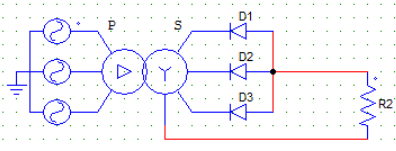Detailed Solution for Test: Three Phase Rectifiers - Question 2

Common anode arrangement because all the anodes are connected to the load side.

Test: Three Phase Rectifiers - Question 3

### In a three-phase half wave diode rectifier using 3 diodes, each diode conducts for

Detailed Solution for Test: Three Phase Rectifiers - Question 3

Each diode conducts for 120 degrees, starting from ωt = 30 degrees.

Test: Three Phase Rectifiers - Question 4

In the below shown diode rectifier circuit,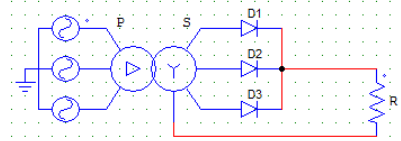The diodes D1, D2 & D3 are connected to phases R,Y and B respectively as shown
The phase sequence is R-Y-B.
The diode D1 would conduct from

Detailed Solution for Test: Three Phase Rectifiers - Question 4

It conducts from 30 to 150, for 90 degrees. D1 starts conducting first as it will be the most positive as it is connected to the R phase.

Test: Three Phase Rectifiers - Question 5

In the below shown diode rectifier circuit,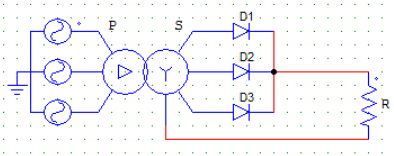The diodes D1, D2 & D3 are connected to phases R,Y and B receptively as shown
The phase sequence is R-Y-B.
The diode D3 conducts from

Detailed Solution for Test: Three Phase Rectifiers - Question 5

It conducts from 270 to 450, for 120 degrees. D1 starts conducting first (from 30 degrees) as it will be the most positive as it is connected to the R phase & likewise.

Test: Three Phase Rectifiers - Question 6

In a three-phase half wave diode rectifier using 3 diodes,

Detailed Solution for Test: Three Phase Rectifiers - Question 6

3 diodes, each conduct for 120 degree at a time.

Test: Three Phase Rectifiers - Question 7

In a three-phase half wave diode rectifier, if Vmp is the maximum phase voltage, then the output voltage on a R load varies from

Detailed Solution for Test: Three Phase Rectifiers - Question 7

The voltage value is positive and varies from (1/2)Vmp to Vmp.

Test: Three Phase Rectifiers - Question 8

The average value of the output voltage, in a 3-phase half wave diode rectifier with Vml as the maximum line voltage value, is given by the expression

Detailed Solution for Test: Three Phase Rectifiers - Question 8

The average value can be obtained by
3 x [ 1/2π x Vml sin ωt d(ωt) ] The integration runs from π/6 to 5π/6 as the diode is conducting for 120 degrees each.

Test: Three Phase Rectifiers - Question 9

In a three-phase half wave 6-pulse mid-point type diode rectifier, each diode conducts for

Detailed Solution for Test: Three Phase Rectifiers - Question 9

In a six-pulse rectifier, each diode conducts once every one cycle, 60° x
6 diodes = 360°.

Test: Three Phase Rectifiers - Question 10

A step-down delta-star transformer, with per-phase turns ration of 5, is fed from a 3-phase, 1100 V, 50 Hz source. The secondary of this transformer through a 3-pulse type rectifier feeds a R load of 10 Ω. Find the maximum value of the load current (phase).

Detailed Solution for Test: Three Phase Rectifiers - Question 10

Vph = 1100/5 = 220 V (Transformer ratio = 5)
Vmp = √2 x 220 V
Imp = Vmp/R.

Test: Three Phase Rectifiers - Question 11

A step-down delta-star transformer, with per-phase turns ratio of 5 is fed from a 3-phase 1100 V, 50 Hz source. The secondary of this transformer is connected through a 3-pulse type rectifier, which is feeding feeding an R load. Find the average value of output voltage.

Detailed Solution for Test: Three Phase Rectifiers - Question 11

Vph = 1100/5 = 220 V (Transformer ratio = 5)
Vmp = √2 x 220 V
Vo = 3√3/2π x Vmp = (√2 x √3 x 3 x 220)/(2 x π).

Test: Three Phase Rectifiers - Question 12

The circuit shown below is that of a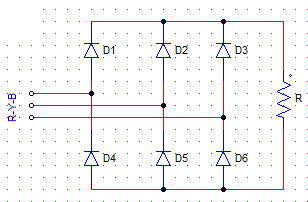Detailed Solution for Test: Three Phase Rectifiers - Question 12

A 3-phase, 6-pulse rectifier consists of 6 diodes connected in 3 legs. Two diodes conduct at a time.

Test: Three Phase Rectifiers - Question 13

A step-down delta-star transformer, with per-phase turns ratio of 5 is fed from a 3-phase 1100 V source. The secondary of this transformer is connected through a 3-pulse type rectifier, which is feeding an R load.
The power delivered to the load is 6839.3 Watts.
The maximum value of the load current is √2 x 22 A.
Fin, the rms value of output voltage Vo (rms)

Detailed Solution for Test: Three Phase Rectifiers - Question 13

Power delivered to the load (Pdc) = Vo(rms)2/R (i)
Imp = Vmp/R
Therefore, R = Vmp/Imp = (1100 x √2)/(5 x √2 x 22) = 10 Ω
Put R in equation (i) & find the required R.M.S voltage.

Test: Three Phase Rectifiers - Question 14

From the diode rectifier circuit shown below, with phase sequence R-Y-B, diodes D3 & D5 conduct when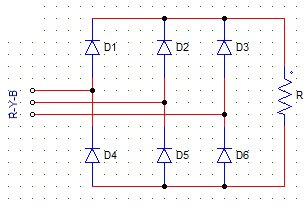Detailed Solution for Test: Three Phase Rectifiers - Question 14

Which diode will conduct depends on where is it in connected? as in in which phase?. D3’s anode is connected to the R phase, hence it will turn on when R is the most positive.

Test: Three Phase Rectifiers - Question 15

From the diode rectifier circuit shown below, with phase sequence R-Y-B, from ωt = 150° to 270°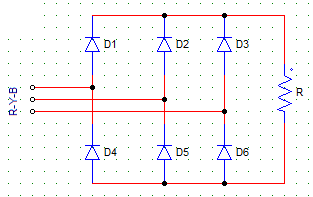Detailed Solution for Test: Three Phase Rectifiers - Question 15

Construct the phase voltage waveforms on a graph. At 150 degree, D2 is forward biased while the other positive group diodes i.e. D2 and D3 remain reserved biased.

Test: Three Phase Rectifiers - Question 16

A 3-phase 6-pulse diode rectifier is shown below with phase sequence R-Y-B. The negative group of diodes (D4, D5, D6) conduct in sequence (from ωt = 0°)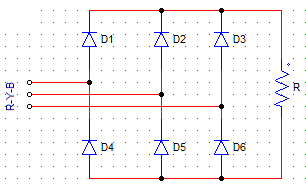Detailed Solution for Test: Three Phase Rectifiers - Question 16

The conduction sequence always depends on the phase sequence, which diode is conducting will depend upon which phase voltage is active at that moment.

Test: Three Phase Rectifiers - Question 17

For a 3-phase 6-pulse diode rectifier, has Vml as the maximum line voltage value on R load. The peak current through each diode is

Detailed Solution for Test: Three Phase Rectifiers - Question 17

Two diodes conduct at a time, constructing the equivalent circuit with supply, R & replacing the conducting diodes by S.C & non-conducting as O.C, the required value can be found out.

Test: Three Phase Rectifiers - Question 18

A 3-phase bridge rectifier, has the average output voltage as 286.48 V. Find the maximum value of line voltage

Detailed Solution for Test: Three Phase Rectifiers - Question 18

Vo = 3Vml/π
Vml = (π x Vo)/3 = 300 V.

Test: Three Phase Rectifiers - Question 19

A 3-phase bridge rectifier charges a 240 V battery. The rectifier is given a 3-phase, 230 V supply. The current limiting resistance in series with the battery is of 8 Ω.
Find the average value of battery charging current.

Detailed Solution for Test: Three Phase Rectifiers - Question 19

Vo = (3√2 x 230)/π = 310.56 V
Draw the battery charging circuit,
Vo = E + (Io x R)
Io = (Vo – E)/R = (310.56 – 240)/8.

Test: Three Phase Rectifiers - Question 20

A 3-phase bridge rectifier charges a 240-V battery. The rectifier is given a 3-phase 230 V supply. The current limiting resistance in series with the battery is 8 Ω.
Find the power delivered to the battery (Pdc).

Detailed Solution for Test: Three Phase Rectifiers - Question 20

Vo = (3√2 x 230)/π = 310.56 V
Draw the battery charging circuit,
Vo = E + (Io x R)
Io = (Vo – E)/R = (310.56 – 240)/8 = 8.82A
Pdc = 240 x 8.82 = 2116 W.

## Power Electronics

5 videos|13 docs|49 tests
 Use Code STAYHOME200 and get INR 200 additional OFF Use Coupon Code
Information about Test: Three Phase Rectifiers Page
In this test you can find the Exam questions for Test: Three Phase Rectifiers solved & explained in the simplest way possible. Besides giving Questions and answers for Test: Three Phase Rectifiers, EduRev gives you an ample number of Online tests for practice

## Power Electronics

5 videos|13 docs|49 tests